## Year 6 resource of the week: factors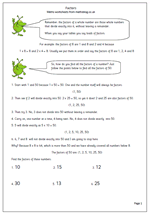Understanding factors and multiples is something many children fail to grasp. Here we have a page on factors and how to find all the factors of a number. The process is fairly straightforward, but can be quite time consuming. A good knowledge of tables and division is also needed. So if children’s knowledge of tables is weak, if they find division difficult and if they have little staying power then it is unlikely that they will enjoy trying to find factors of numbers!

One point that even brighter children take a while to understand is that you only need to continue to divide up to when the number squared is larger than the original number eg to find the factors of 62 you only need to divide 62 by numbers up to 7 because eight eights are 64. It is a good idea to spend some time explaining and showing the logic of this to children.

This can be found in our Year 6 Understanding Number section.

Factors 1

## Single step word problems for year 6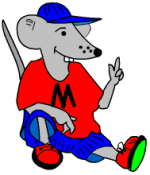Below are two pages of maths problems written in words. They are known as ‘single step operations’ as only one mathematical process is necessary to solve them. Children find word problems very difficult, but the one step type are much, much easier than the two step.Children need to be able to read and understand problems written in prose that include elements of real life, either at home or at school. They need to be able to see what processes are necessary to solve it and then lay out their answer clearly, giving some explanation. If they have had plenty of practice at writing their own number stories in earlier years they will now find these much easier.

Single step operations (pg 1)

Single step operations (pg 2)

## Resource of the Week: interpret pie charts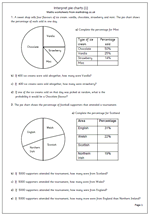Something which looksvery easy, but can cause difficulties for this week’s Resource of the Week. Pie charts are a good way to illustrate the proportion of a whole amount or quantity. The arc length of each sector or the sectors area is proportional to the quantity it represents. This might sound a little tricky, but pie charts can be effective in displaying information.

This worksheet looks at a pie chart where the percentages have also been given. This allows for numbers to be worked out if the total number is given. The first pie chart looks at ice cream sales and the second looks at football supporters attending a tournament.

Pie chart (1)

## Year 6 money problems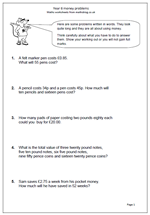There are not many questions on this page but they are tricky with some requiring several stages to answer. They are all to do with money and involve addition, multiplication and division.

As a preview to answering SAT questions this page asks for all working out to be shown and tips on the answer page will help decide whether an extra mark can be given for showing suitable working out, even if the final answer is incorrect. (Be very suspicious of any answers which are written down without showing any working as they may well have been answered using a calculator.)

Money problems

## Resource of the Week: Maths SAT questions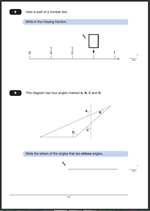For anyone in Year 6 the Maths SAT tests will be coming up before too long! Don’t forget that we have a whole section of past questions and answers, including great explanations of the best ways to approach each question. Invaluable help to achieve those higher levels!

This week I am highlighting are two questions from the Maths SAT Paper 2011 Paper B. The first involves equivalent fractions and the second angles and shape.

The answer to Question 8.   3/4
One mark awarded for a correct answer.
Equivalent fractions accepted as is 0.75.
Suggested method:
This question is another reading a number line problem and if children can count in quarters it is an easy mark. Firstly, it has to be recognised that the number line is showing quarters (1/2 is equivalent to 2/4 and 1 is equivalent to 4/4) making the missing number 3/4.

The answer to Question 9. A and D.
One mark awarded for a correct answer.
The letters can be given in either order. Both need to be given with no incorrect angles added.
Suggested method:
Answering this question correctly depends on 3 things:
Firstly, a recognition of the conventions for labelling angles, using the arc.
Secondly, a knowledge that an obtuse angle is larger than 90 degrees but less than 180 degrees.
If either of these are unknown it becomes purely a guessing game. (Some children do think that the angle depends on the length of the lines rather than a measure of turn.)

Thirdly, if these are known, then the obtuse angles still need to be recognised. One way to do this is to slide a right angle (eg a corner of a piece of paper) into the angle to see whether it is smaller or larger.

Why not visit ks2-maths-sats.co.uk for free SATs papers and a great SAT revision programme?

Questions 8 and 9 from SAT Paper B 2011

Questions 8 and 9 answers and suggested method 2011 Paper B

## 12.12.12 an interesting date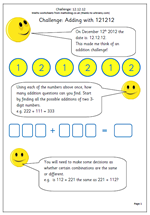Those of you who have followed the site for some time will know that I enjoy the quirky dates which come up from time to time, and we have a really good one next week; on 12th December 2012 the date can be written as 12.12.12. On the 12th second of the 12th minute of the 12th hour it can be written as 12.12.12.12.12.12!!

It will be quite some time before this type of recurring number pattern happens again; in fact not until 2101, when I don’t think I will be around to enjoy it!!

of course there are the usual doom mongers suggesting that the world will end as we all get sucked into a black hole, but I think it is a great chance to do a little maths and I was delighted to see that urbrainy.com have published a superb couple of worksheets which they have allowed me to publish.

The first takes a look at the digits 121212 and how many different 3-digit addition sums can be made from them. This type of activity really encourages thinking in a logical, well ordered way and it also brings some issues up, in particular whether the order of addition makes any difference as is 111 + 222 the same as 222 + 111. This is suitable for children around year 4 and can be found in the Year 4 Using and Applying Maths category.

The second set of worksheets looks at using three twelves and the four operations and raises all kinds of interesting mathematical questions. Suitable for Year 6 who have been introduced to BODMAS or as an introduction to it.

Calculating with 12 12 12

## Year 6: solve problems involving money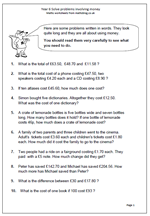By year 6 children should be able to read and understand problems written in prose that include some elements of real life involving money.

They should be able to read the problem, understand the situation described, be able to see what processes are needed to solve it and then lay out working and answers clearly.

Children do find word problems far harder than just being given a ‘sum’ to do and it is important to discuss with them the best way to lay out any working.

One or two of these questions could be done mentally, but most need more than one process to reach the answer. All involve money and are an excellent way of working with two places after the decimal point.

Solve word problems involving money

## Key Stage 2 SAT questions on time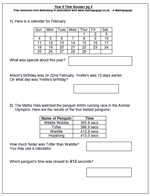Time questions are very popular with the SAT test writers and this page shows some typical examples, using tables and charts.

Children often get confused by questions which say that an event was earlier or later when looking at a calendar. If it was earlier then the number of days have to be subracted. On a calendar moving up one position will subtract 7 days – useful when counting back.

Many more time questions can be found in the year 6 category, under Key Stage 2 Maths SAT Practice.
KS2 SAT maths worksheet: Time (p 4)

## Resource of the week: KS2 SAT style questions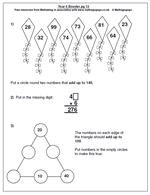It’s never too early to get to grips with some SAT style questions in Year 6 and we have an increasing number of these. There are two similar maths worksheets here which demonstrate a number of techniques that can be used to answer SAT questions.

The first question asks for two numbers which add up to 140 from the selection given. The easiest approach to this is firstly to look at the units and find two which add up to 10, such as 28 and 32. Then to look to see if the tens digits are suitable; in this case they are not.

Try again 99 and 91 – this time too big.

Try 74 and 66.

6 and 4 make 10. 70 and 60 make 130. Add 10 to 130 makes 140 – there is your answer!

For question 3 the order is important. Start with an edge of the triangle which already has two numbers in.

Booster maths worksheet 13

Booster maths worksheet 14

## Square numbers and square roots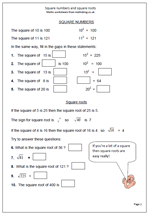A square number is a whole number multiplied by itself for example 3 x 3 = 9. This makes 9 a square number. By Year 6 most children will be familiar with square numbers and hopefully will know the first ten or so square numbers off be heart as they all appear in the times tables.

The square root of a number is a value, or number, that can be multiplied by itself to give the original number.

For example: the square root of 16 is 4 because 4 x 4 = 16

The square root of a number is usually written using the ‘tick’ square root sign. Each whole number is the square root of another number:

5 is the square root of 25

6 is the square root of 36 etc

This page takes a quick look at square numbers and square roots and can be found in our Year 6, Knowing Number facts section.

Square numbers and square roots